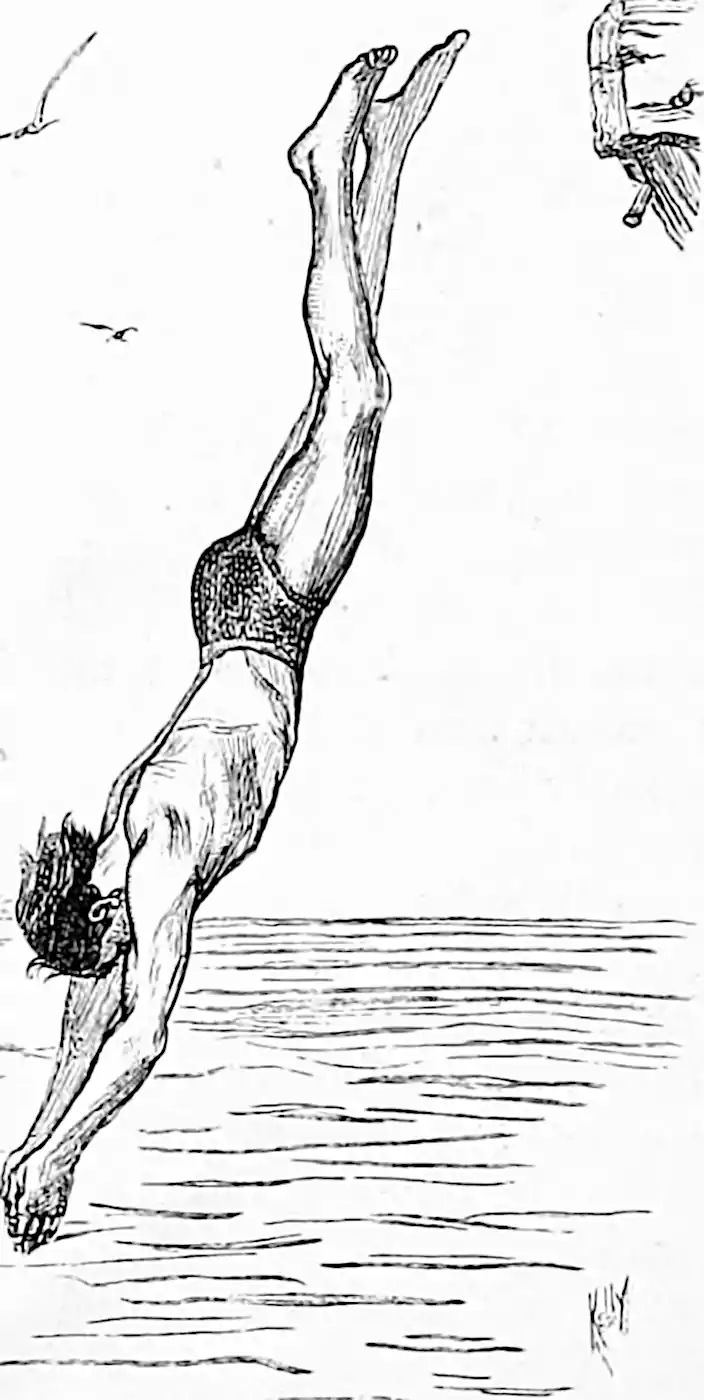$\renewcommand{\var}{\operatorname{Var}} \renewcommand{\dd}{\mathrm{d}} \renewcommand{\bb}{\mathbb{#1}} \renewcommand{\vv}{\boldsymbol{#1}} \renewcommand{\rv}{\mathsf{#1}} \renewcommand{\vrv}{\vv{\rv{#1}}} \renewcommand{\gvn}{\mid} \renewcommand{\Ex}{\mathbb{E}} \renewcommand{\Pr}{\mathbb{P}}$Stochastic Newton-type optimization, unlike deterministic Newton optimisation, uses noisy (possibly approximate) 2nd-order gradient information to find the argument which minimises

$x^*=\operatorname{arg min}_{\mathbf{x}} f(x)$

for some an objective function $$f:\mathbb{R}^n\to\mathbb{R}$$.

## Subsampling data

Most of the good tricks here are set up for ML-style training losses where the bottleneck is summing a large number of loss functions.

LiSSA attempts to make 2nd order gradient descent methods scale to large parameter sets :

a linear time stochastic second order algorithm that achieves linear convergence for typical problems in machine learning while still maintaining run-times theoretically comparable to state-of-the-art first order algorithms. This relies heavily on the special structure of the optimization problem that allows our unbiased hessian estimator to be implemented efficiently, using only vector-vector products.

David McAllester observes:

Since $$H^{t+1}y^t$$ can be computed efficiently whenever we can run backpropagation, the conditions under which the LiSSA algorithm can be run are actually much more general than the paper suggests. Backpropagation can be run on essentially any natural loss function.

uses a decomposition of the objective into a sum of simple functions (the classic SGD setup for neural nets, typical of an online optimisation.

What do get us?

Many machine learning and signal processing problems are traditionally cast as convex optimization problems. A common difficulty in solving these problems is the size of the data, where there are many observations ('large n') and each of these is large ('large p'). In this setting, online algorithms such as stochastic gradient descent which pass over the data only once, are usually preferred over batch algorithms, which require multiple passes over the data. In this talk, I will show how the smoothness of loss functions may be used to design novel algorithms with improved behavior, both in theory and practice: in the ideal infinite-data setting, an efficient novel Newton-based stochastic approximation algorithm leads to a convergence rate of O(1/n) without strong convexity assumptions, while in the practical finite-data setting, an appropriate combination of batch and online algorithms leads to unexpected behaviors, such as a linear convergence rate for strongly convex problems, with an iteration cost similar to stochastic gradient descent. (joint work with Nicolas Le Roux, Eric Moulines and Mark Schmidt).

## General case

Rather than observing $$\nabla f, \nabla^2 f$$ we observe some random variables $$G(x),H(x)$$ with $$\bb{E}G=\nabla f$$ and $$\bb{E}(H)=\nabla^2 f,$$ not necessarily decomposable into a sum.

🏗

## Online Newton’s method

Bookmarked: I am sitting in Iman Shames’ seminar on some recent papers :

In this talk we revisit one of the most important workhorses of numerical optimisation: the Newton’s method. We will (hopefully) provide some insight into the role it still plays in modern applications of control and learning theory. We first look into its regret analysis when applied to online nonconvex optimisation problems, then we shift our focus to how it and its very close relative, the midpoint Newton’s method, play leading roles in learning dynamical systems, and conclude with reviewing how it acts as a hero in disguise in solving differential dynamic programming problems.

Looks worthwhile.

Online Newton’s Method (ONM): $$\quad x_{t+1}=x_{t}-H_{t}^{-1}\left(x_{t}\right) \nabla f_{t}\left(x_{t}\right)$$

Let $$x_{t+1}^{*}=x_{t}^{*}+v_{t}$$ with $$\max _{t}\left\|v_{t}\right\| \leq \bar{v}$$ and $$V_{T}=\sum_{t=1}^{T}\left\|x_{t+1}^{*}-x_{t}^{*}\right\|=\sum_{t=1}^{T}\left\|v_{t}\right\|$$ Some Assumptions \begin{aligned} \exists h_{t}>0: &\left\|H_{t}^{-1}\left(x_{t}^{*}\right)\right\| \leq \frac{1}{h_{t}} \\ \exists \beta_{t}, L_{t}>0: &\left\|x-x_{t}^{*}\right\| \leq \beta_{t}=\left\|H_{t}(x)-H_{t}\left(x_{t}^{*}\right)\right\| \leq L_{t}\left\|x-x_{t}^{*}\right\| \\ \left\|x_{t}-x_{t}^{*}\right\| & \leq \gamma_{t}=\min \left[\beta_{t}, \frac{2 h_{t}}{3 L_{t}}\right] \end{aligned} Lemma $\left((\mathrm{ONM}) \wedge\left(\bar{v} \leq \gamma-\frac{3 L}{2 h} \gamma^{2}\right)\right) \Longrightarrow\left(\left\|x_{t+1}-x_{t+1}^{*}\right\|<\gamma\right)$ Another assumption: $$\exists \ell>0$$ such that $$\left\|x-x_{t}^{*}\right\| \leq \gamma \Longrightarrow\left|f_{t}(x)-f_{t}\left(x_{t}^{*}\right)\right| \leq\left\|x-x_{t}^{*}\right\|$$ for all $$t=1,2, \ldots, T$$. Theorem The regret of ONM is bounded above: $\operatorname{Reg}_{T}^{D, 0 N M} \leq \frac{\ell}{1-\frac{3 L}{2 h} \gamma}\left(V_{T}+\delta\right)$ where $$\delta=\frac{3 L}{2 h}\left(\left\|x_{0}-x_{0}^{*}\right\|^{2}-\left\|x_{T}-x_{T}^{*}\right\|^{2}\right)$$

It is the same as the regret for strongly-convex functions.

Take-home message seems to be Newton’s methods are efficient for sufficiently online control problems rather as you would expect. It is not clear to me that getting a decent Quasi-Newton method is clear or obvious?

## Subsampling parameters

Hu et al. (2022):

The success of deep learning comes at a tremendous computational and energy cost, and the scalability of training massively overparametrized neural networks is convergence rate in non-convex settings, both in theory and practice.

To mitigate this cost, recent works have proposed to employ alternative (Newton-type) training methods with much faster convergence rate, albeit with higher cost-periteration. For a typical neural network with $$m=\operatorname{poly}(n)$$ parameters and input batch of $$n$$ datapoints in $$\mathbb{R}^{d}$$, the previous work of [Brand, Peng, Song, and Weinstein, ITCS'2021] requires $$\sim m n d+n^{3}$$ time per iteration. In this paper, we present a novel training method that requires only $$m^{1-\alpha} n d+n^{3}$$ amortized time in the same DNNs. This method relies on a new and alternative view of neural networks, as a set of binary search trees, where each iteration corresponds to modifying a small subset of the nodes in the tree. We believe this view would have further applications in the design and analysis of DNNs.

### No comments yet. Why not leave one?

GitHub-flavored Markdown & a sane subset of HTML is supported.